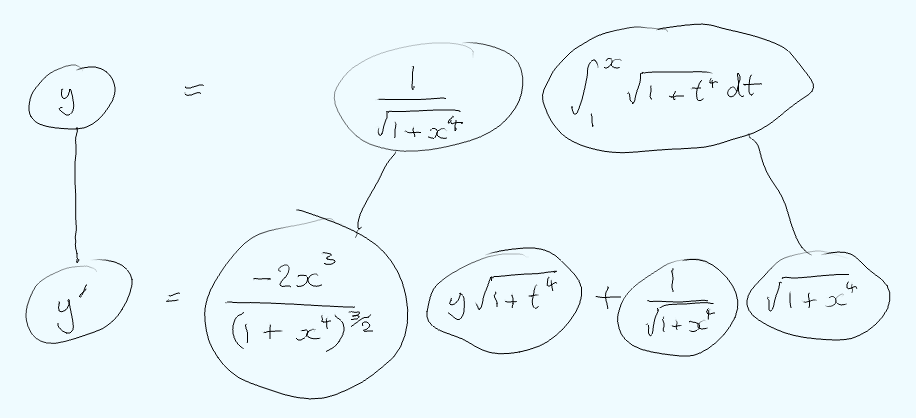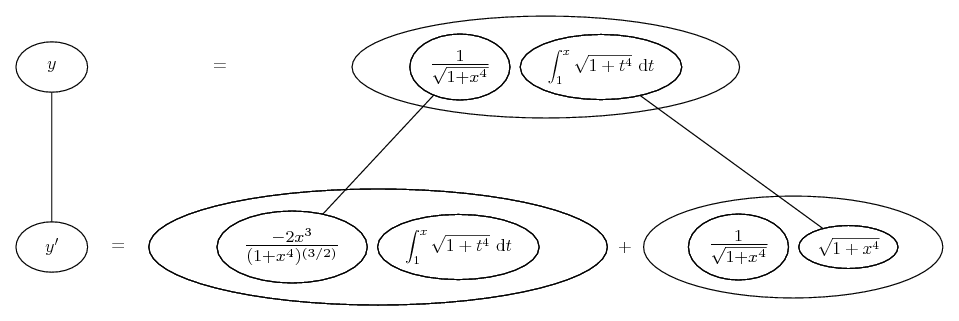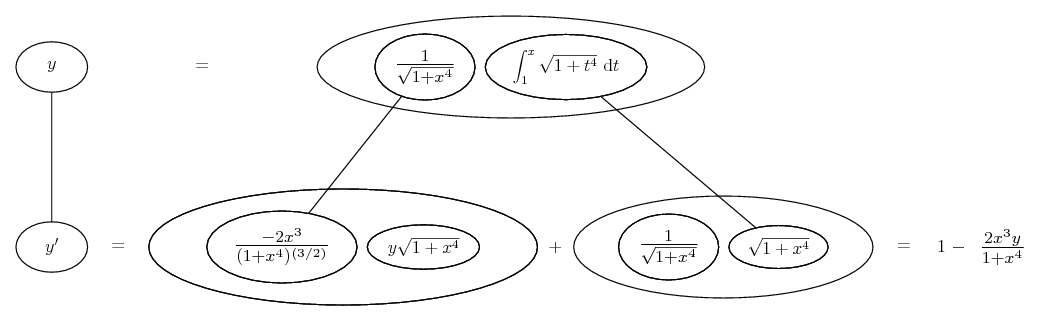# Thread: Verifying Differential Equation

1. ## Verifying Differential Equation

The question is read so that y=f(x) is a solution of the given differntial equation

where y= 1/sqrt(1+x^4)* int (1 to x) sqrt(1+t^4)dt and
y prime+((2x^3)(y))/(1+x^4)

I tried solving the integral from 1 to x using substitution u=1+t^4 but i still cant get it... please help me

2.Originally Posted by dreamx20y prime+((2x^3)(y))/(1+x^4)
Should that be y prime = 1 + the rest?

Though, I'm getting y prime = 1 - the rest. Anyway, just in case a picture helps...... where...... is the product rule. Straight continuous lines differentiate downwards (integrate up) with respect to x. The right-hand differentiation uses the FTC.

Edit: here's a neater pic...Then, writing the lower integral as the solution for the top one in the top row..._________________________________________

Don't integrate - balloontegrate!

Balloon Calculus; standard integrals, derivatives and methods

Balloon Calculus Drawing with LaTeX and Asymptote!

#### Search Tags

differential, differential equation, equation, verifying